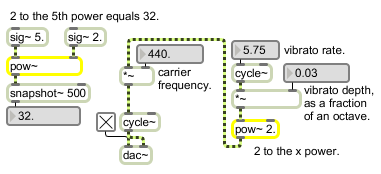# pow~

Signal power function

## Description

pow~ raises the base value (set in the right inlet) to the power of the exponent (set in the left inlet). Either inlet can receive a signal, float or int.

## Arguments

Name Type Opt Description
base-value float or int opt Sets the base value. The default value is 0. If a signal is connected to the right inlet, the argument is ignored.

## Messages

 float exponent/base-value [float] In left inlet: Sets the exponent. If there is a signal connected to the left inlet, a number received in the left inlet is ignored. In right inlet: Sets the base value. If there is a signal connected to the right inlet, a number received in the right inlet is ignored. signal In left inlet: Sets the exponent. In right inlet: Sets the base value.

## Output

signal: The base value (from the right inlet) raised to the exponent (from the left inlet).

## ExamplesComputes the mathematical expression xy for converting to logarithmic or exponential scale